# What Is Calculus? A Beginner's Guide to Limits and Differentiation

Eugene is a qualified control/instrumentation engineer Bsc (Eng) and has worked as a developer of electronics & software for SCADA systems.

## How to Understand Calculus

Calculus is a study of rates of change of functions and accumulation of infinitesimally small quantities. It can be broadly divided into two branches:

• Differential Calculus. This concerns rates of changes of quantities and slopes of curves or surfaces in 2D or multidimensional space.
• Integral Calculus. This involves summing infinitesimally small quantities.

## What's Covered in This Tutorial

In this tutorial, you will learn about:

• Limits of a function
• How the derivative of a function is derived
• Rules of differentiation
• Derivatives of common functions
• What the derivative of a function means
• Working out derivatives from first principles
• 2nd and higher order derivatives
• Applications of differential calculus
• Worked examples

## Who Invented Calculus?

Calculus was invented by the English mathematician, physicist and astronomer Isaac Newton and German mathematician Gottfried Wilhelm Leibniz independently of each other in the 17th century.Isaac Newton (1642 - 1726) and Gottfried Wilhelm Leibniz (below) invented calculus independent of each other in the 17th century.https://pixabay.com/vectors/isaac-newton-portrait-vintage-3936704/Gottfried Wilhelm von Leibniz (1646 - 1716), a German philosopher and mathematicianPublic domain image via Wikipedia.

## What Is Calculus Used For?

Calculus is used widely in mathematics, science, in the various fields of engineering and economics.

## Introduction to Limits of Functions

To understand calculus, we first need to grasp the concept of limits of a function.

Imagine we have a continuous line function with the equation f(x) = x + 1 as in the graph below.

The value of f(x) is simply the value of the x coordinate plus 1.

The function is continuous which means that f(x) has a value that corresponds to all values of x, not just the integers ....-2, -1, 0, 1, 2, 3.... and so on, but all the intervening real numbers. I.e. decimals numbers like 7.23452, and irrational numbers like π, and √3.

So if x = 0, f(x) = 1

Scroll to Continue

## Read More From Owlcation

if x = 2, f(x) = 3

if x = 2.3, f(x) = 3.3

if x = 3.1, f(x) = 4.1 and so on.

Let's concentrate on the value x =3, f(x) = 4.

As x gets closer and closer to 3, f(x) gets closer and closer to 4.

So we could make x = 2.999999 and f(x) would be 3.999999.

We can make f(x) as close to 4 as we want. In fact we can choose any arbitrarily small difference between f(x) and 4 and there will be a correspondingly small difference between x and 3. But there will always be a smaller distance between x and 3 that produces a value of f(x) closer to 4.

## So What's the Limit of a Function Then?

Referring to the graph again, the limit of f(x) at x = 3 is the value f(x) approaches as x gets closer to 3. Not the value of f(x) at x=3, but the value it approaches. As we'll see later, the value of a function f(x) may not exist at a certain value of x, or it may be undefined.This is expressed as, "The limit of f(x) as x approaches c, equals L".© Eugene Brennan

## Formal Definition of a Limit

### The (ε, δ) Cauchy Definition of a Limit

The formal definition of a limit was specified by the mathematicians Augustin-Louis Cauchy and Karl Weierstrass

Let f(x) be a function defined on a subset D of the real numbers R.

c is a point of the set D. ( The value of f(x) at x = c may not necessarily exist)

L is a real number.

Then:

lim f(x) = L
x → c

exists if:

• Firstly for every arbritarily small distance ε > 0 there exists a value δ such that, for all x belonging to D and 0 > | x - c | < δ, then | f(x) - L | < ε
• and secondly the limit approaching from the left and right of the x coordinate of interest must be equal.

In plain English, this says that the limit of f(x) as x approaches c is L, if for every ε greater than 0, there exists a value δ, such that values of x within a range of c ± δ (excluding c itself, c + δ and c - δ) produces a value of f(x) within L± ε.

....in other words we can make f(x) as close to L as we want by making x sufficiently close to c.

This definition is known as a deleted limit because the limit omits the point x = c.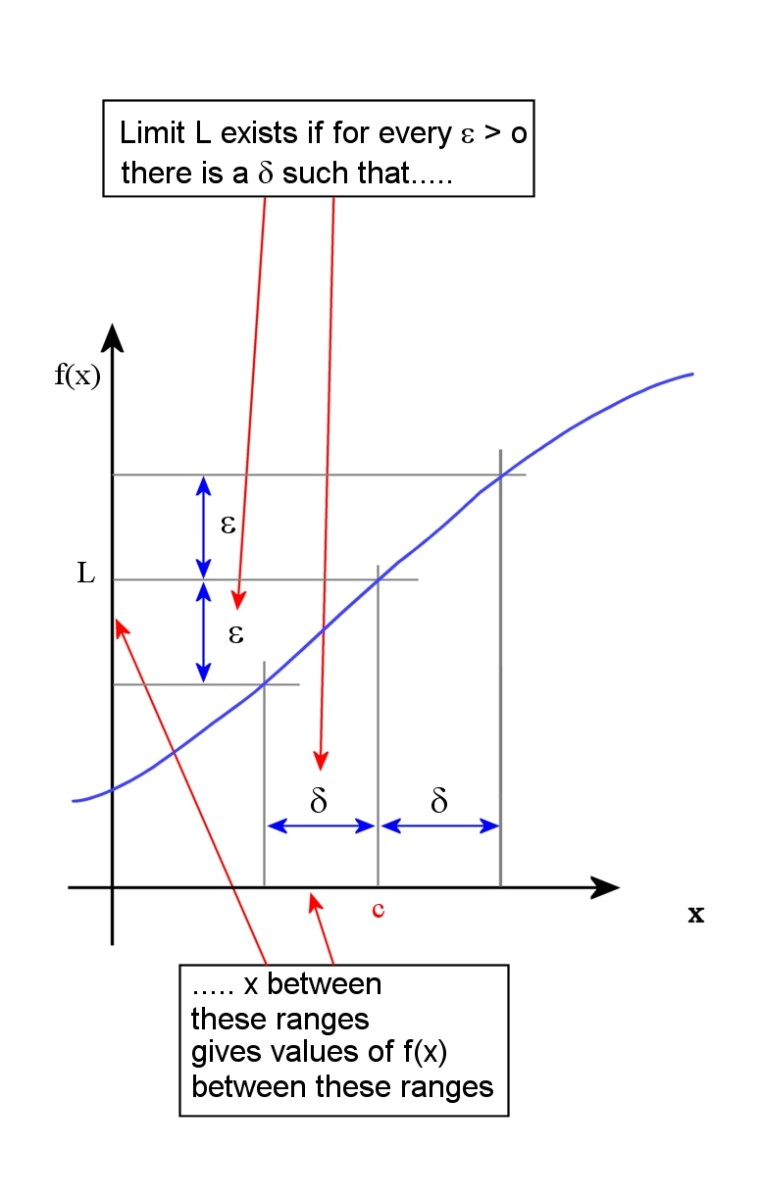Limit of a function. 0 > |x - c| then 0 > | f(x) - L | < ϵ© Eugene Brennan

## Continuous and Discontinuous Functions

A function is continuous at a point x = c on the real line if it is defined at c and the limit equals the value of f(x) at x = c. I.e:

lim f(x) = L = f(c)
x → c

A continuous function f(x) is a function that is continuous at every point over a specified interval.

Examples of continuous functions:

• Temperature in a room versus time.
• The speed of a car as it changes over time.

A function that is not continuous, is said to be discontinuous. Examples of discontinuous functions are:

• Your bank balance. It changes instantly as you lodge or withdraw money.
• A digital signal, it's either 1 or 0 and never in between these values.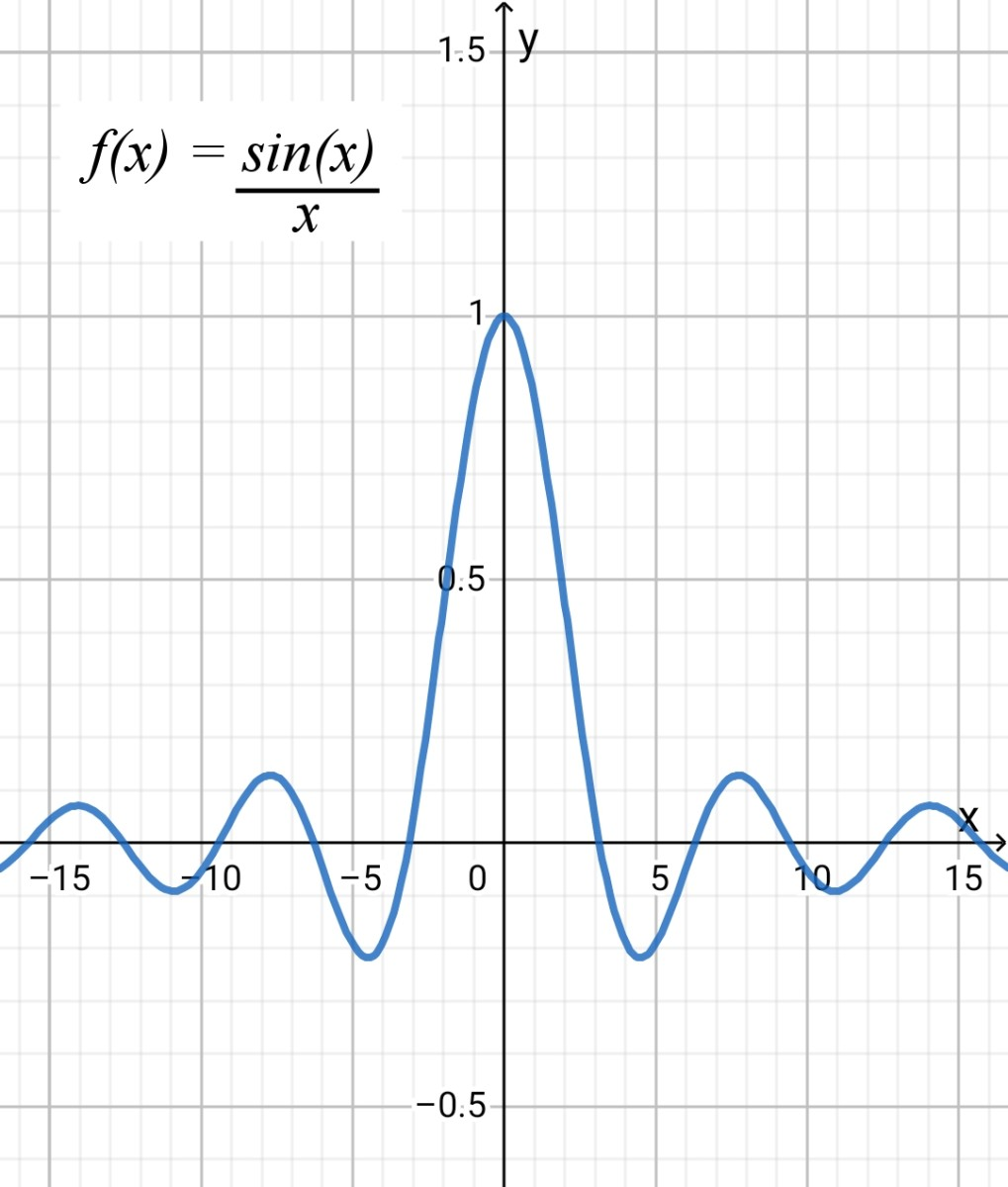The function f(x) = sin (x) / x or sinc (x). The limit of f(x) as x approaches 0 from both sides is 1. The value of sinc (x) at x = 0 is undefined because we can't divide by zero and sinc (x) is discontinuous at this point.© Eugene Brennan

## Limits of Common Functions

FunctionLimit

1/x as x tends to infinity

0

a/(a + x) as x tends to 0

a

sin x/x as x tends to 0

1

## Calculating the Velocity of a Vehicle

Imagine we record the distance a car travels over a period of one hour. Next we plot all the points and join the dots, drawing a graph of the results (as shown below). On the horizontal axis, we have the time in minutes and on the vertical axis we have the distance in miles. Time is the independent variable and distance is the dependent variable. In other words, the distance travelled by the car depends on the time which has passed.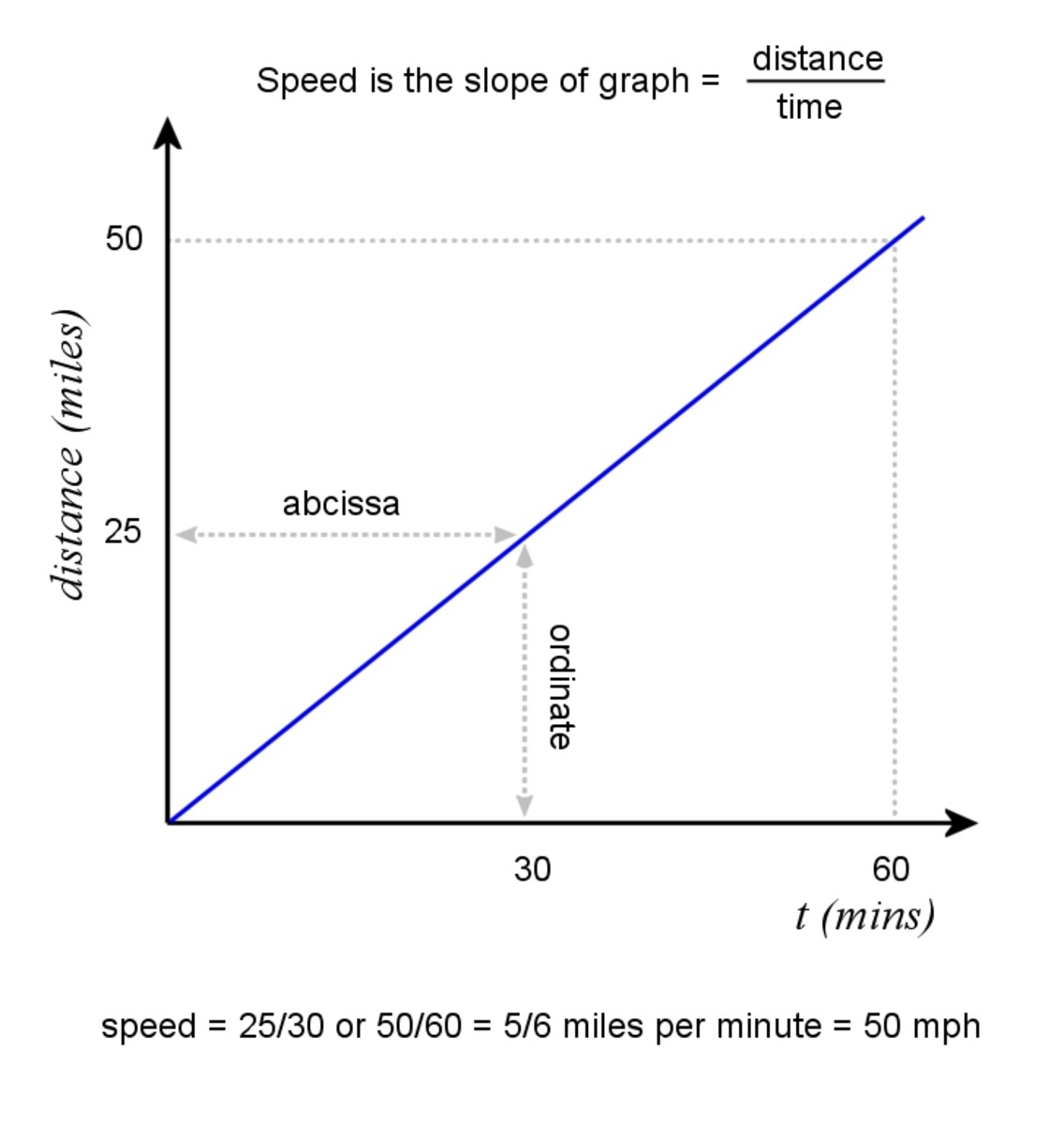Graph of distance travelled by a vehicle at constant speed is a straight line.© Eugene Brennan

If the car travels at a constant velocity, the graph will be a line, and we can easily work out its velocity by calculating the slope or gradient of the graph. To do this in the simple case where the line passes through the origin, we divide the ordinate (vertical distance from a point on the line to the origin) by the abscissa (horizontal distance from a point on the line to the origin).

So if it travels 25 miles in 30 minutes,

Velocity = 25 miles/30 minutes = 25 miles / 0.5 hour = 50 mph

Similarly if we take the point at which it has travelled 50 miles, the time is 60 minutes, so:

Velocity is 50 miles/60 minutes = 50 miles / 1 hour = 50 mph

Note: In physics we normally speak of the "velocity" of a body. Technically, the definition of velocity is speed in a given direction, so it is a vector quantity. Speed is the magnitude of the velocity vector.

## Average Velocity and Instantaneous Velocity

Ok, so this is all fine if the vehicle is travelling at a steady velocity. We just divide distance by time taken to get velocity. But this is the average velocity over the 50 mile journey. Imagine if the vehicle was speeding up and slowing down as in the graph below. Dividing distance by time still gives the average velocity over the journey, but not the instantaneous velocity which changes continuously. In the new graph, the vehicle accelerates mid way through the journey and travels a much greater distance in a short period of time before slowing down again. Over this period, its velocity is much higher.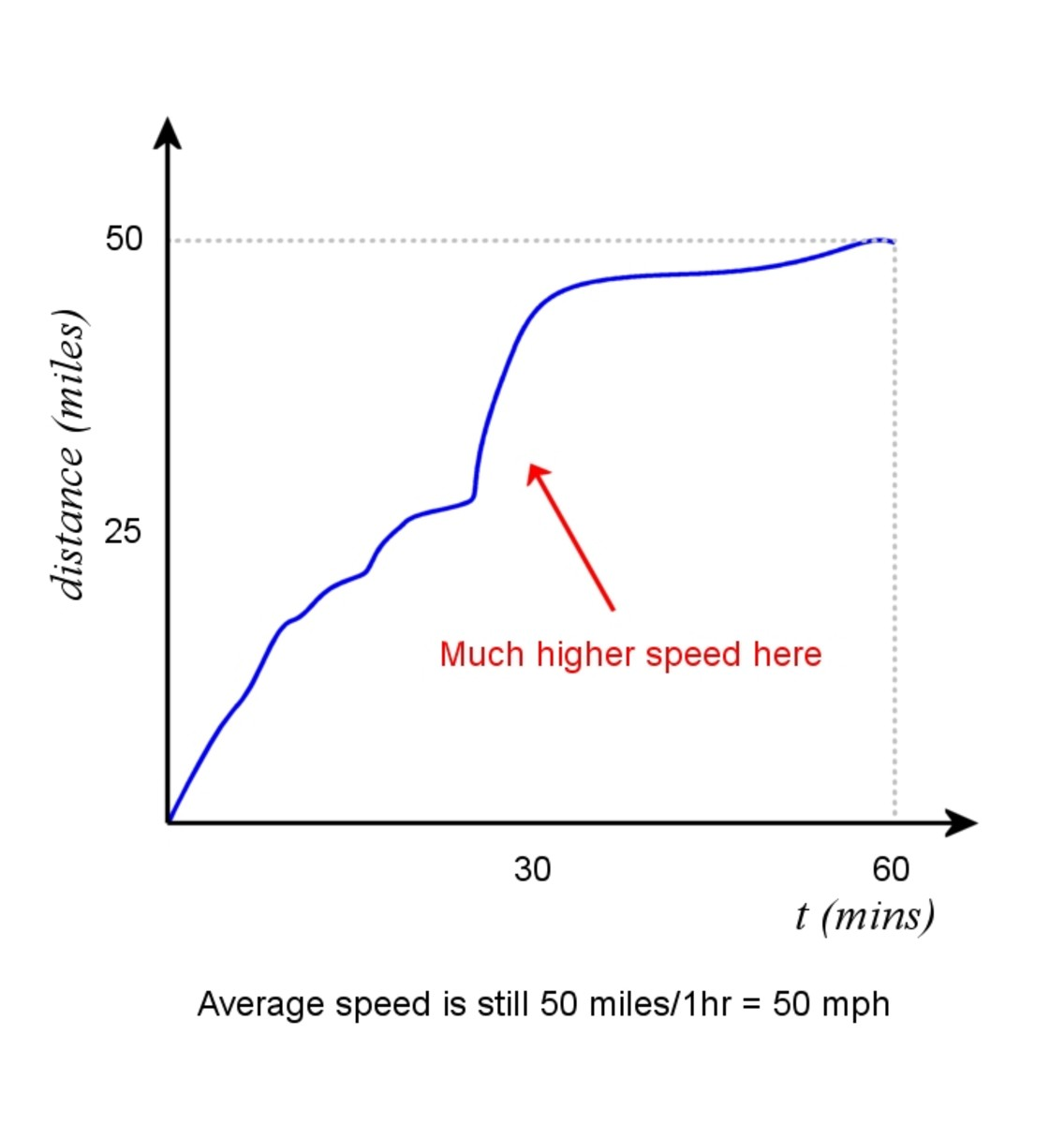Graph of a vehicle travelling at a variable speed© Eugene Brennan

In the graph below, if we denote the small distance travelled by Δs and the time taken as Δt, again we can calculate velocity over this distance by working out the slope of this section of the graph.

So average velocity over interval Δt = slope of graph = Δs/Δt

Note: The Greek letter "Δ" pronounced "delta" is often used in mathematics to represent a small quantity.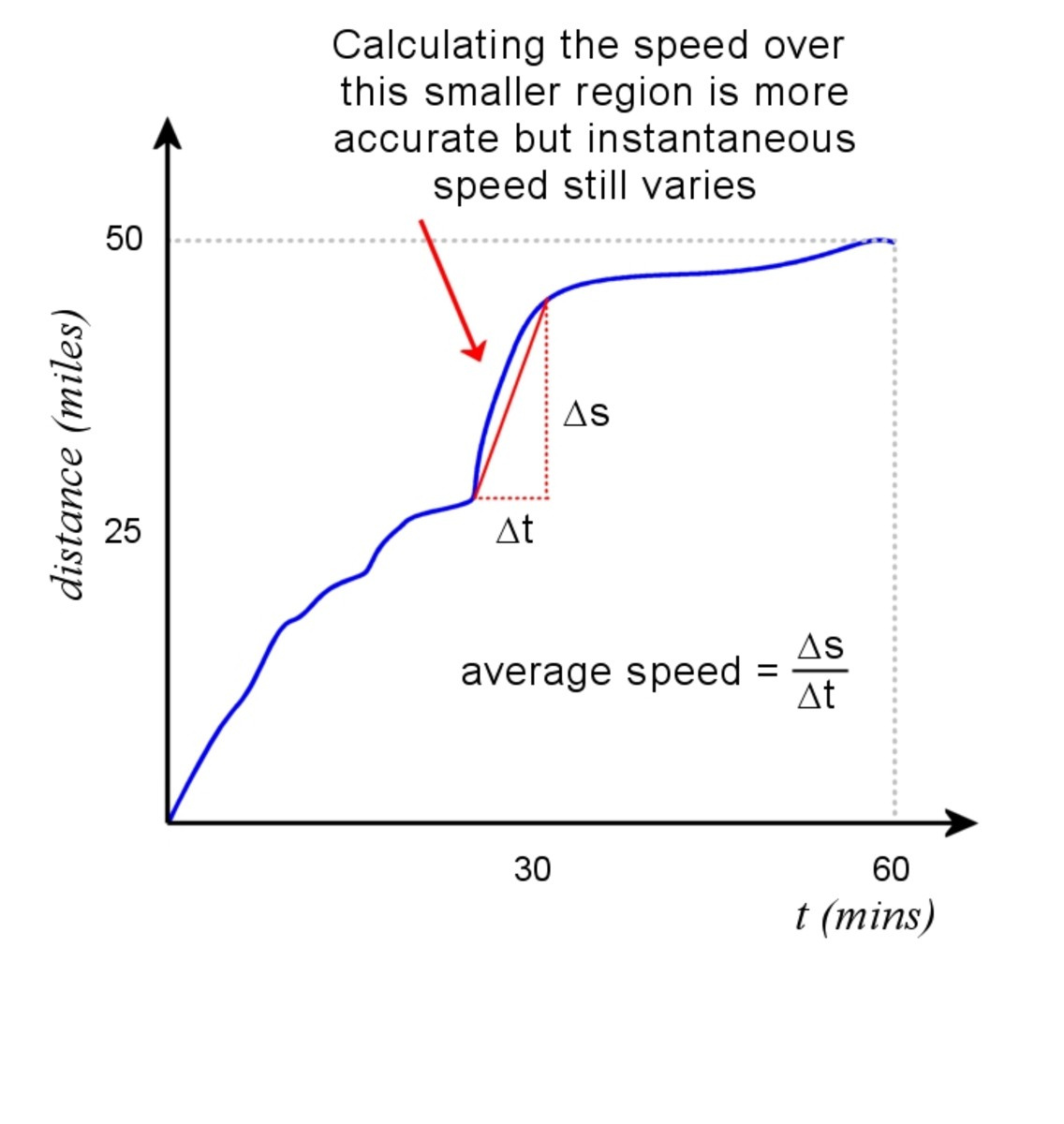Approximate speed over a short range can be determined from slope. The average speed over the interval Δt is Δs/Δt.© Eugene Brennan

However the problem is that this still only gives us an average. It's more accurate than working out velocity over the full hour, but it's still not the instantaneous velocity. The car travels faster at the start of the interval Δt (we know this because distance changes more rapidly and the graph is steeper). Then the velocity starts to decrease midway and reduces all the way to the end of the interval Δt.

What we're aiming to do is find a way of determining the instantaneous velocity.
We can do this by making Δs and Δt smaller and smaller so we can work out the instantaneous velocity at any point on the graph.

See where this is heading? We're going to use the concept of limits we learned about before.

## What Is Differential Calculus?

Differential calculus is one of the two branches of calculus which also includes integral calculus. It is a study of the rate at which quantities change.

In the example above we saw how we could attempt to determine a more accurate measurement of velocity by working out the slope of a graph over a shorter interval. We can do this using limits.

### Slope of a graph

In the graph below we have a generalised function y = f (x).

x is a point on the horizontal axis and Δx is a small change in x.

The value of the function at x is f (x)

As x changes to x + Δx , f (x) changes by Δy to f (x + Δx)

You may remember from coordinate geometry if we know two points on a graph: (x1, y1) and (x2, y2), the slope of a line joining the two points is:

(y2 - y1) / ( x2 - x1)

So the slope of this line is ( f (x + Δx) - f (x) ) / (x + Δx - x) = Δy / Δx

The slope Δy / Δx is approximately the slope of a tangent to the graph for small Δx.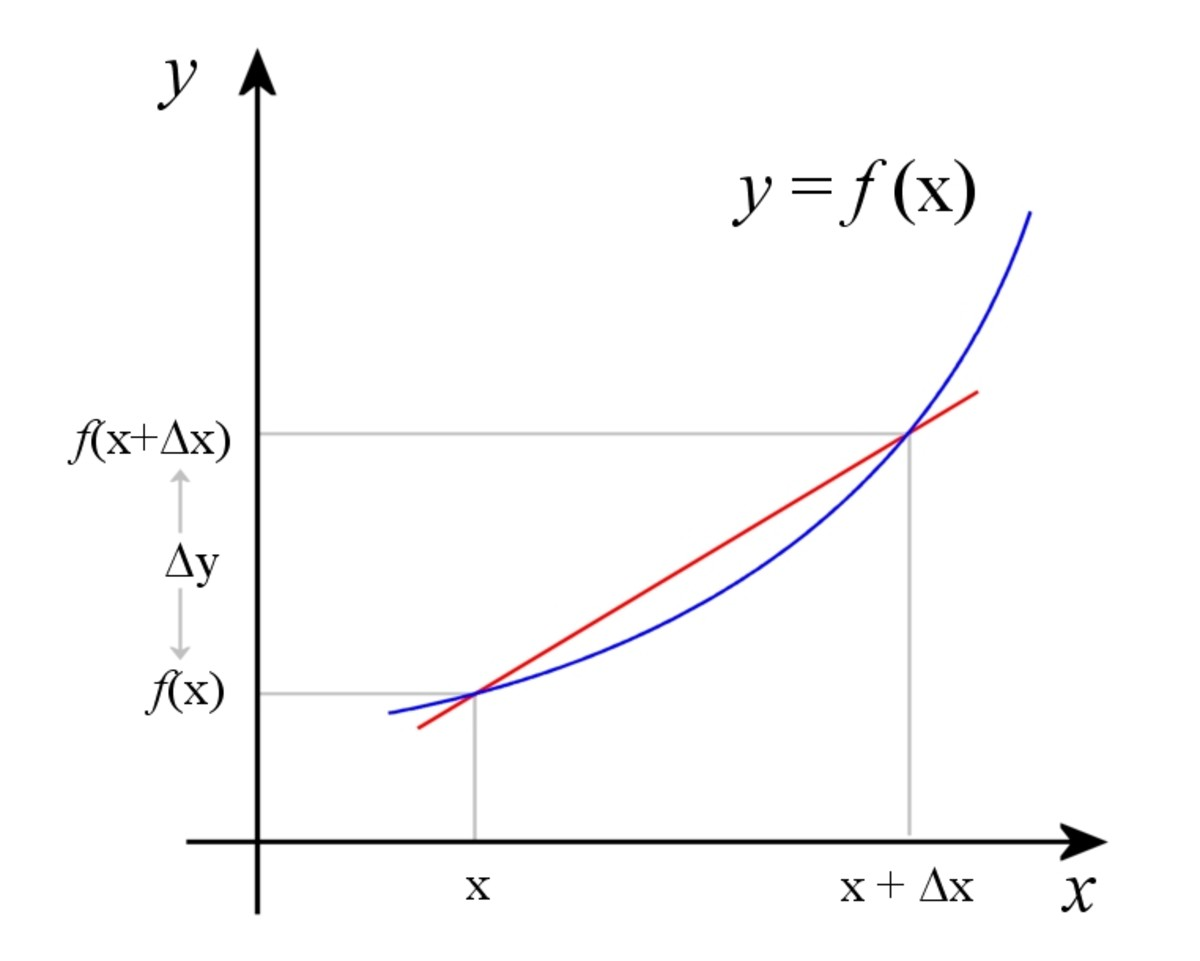Over a short distance, the slope of the secant (red line) is approximately the rate of change of the function.© Eugene Brennan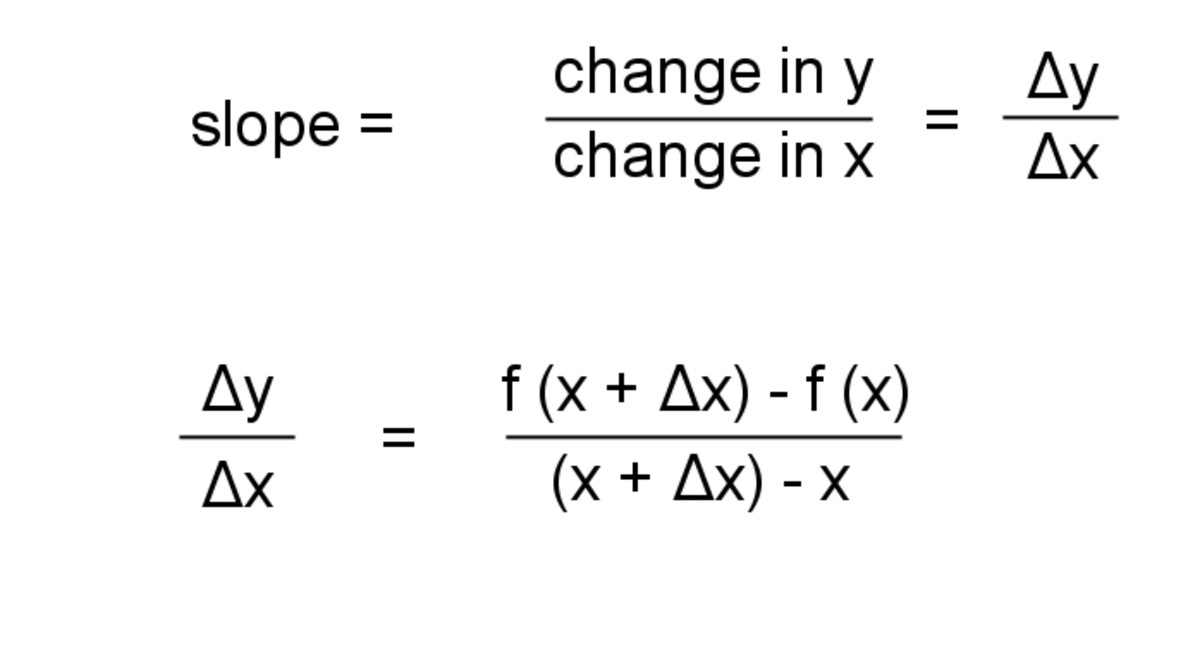Approximate slope of a function for small increments of x and f(x)© Eugene Brennan

## What Happens When ΔX Becomes Smaller and Smaller?

The red line that intersects the graph at two points in the diagram above is called a secant.

If we now make Δx and Δy smaller and smaller, the red line eventually becomes a tangent to the curve. The slope of the tangent is the instantaneous rate of change of f (x) at the point x.

### Derivative of a Function

If we take the limit of the value of the slope as Δx tends to zero, the result is called the derivative of y = f (x).

lim (Δy / Δx) =
Δx → 0

= lim ( f (x + Δx) - f (x) ) / (x + Δx - x)
Δx → 0

The value of this limit is denoted as dy/dx.

Since y is a function of x, i.e. y = f(x), the derivative dy/dx can also be denoted as f '(x) or just f ' and is also a function of x. I.e. it varies as x changes.

If the independent variable is time, the derivative is sometimes denoted by the variable with a dot superimposed on top.

E.g. if a variable x represents position and x is a function of time. I.e. x(t)

Derivative of x wrt t is dx/dt or ( or dx/dt is speed, the rate of change of position)

We can also denote the derivative of f (x) wrt x as d/dx(f (x))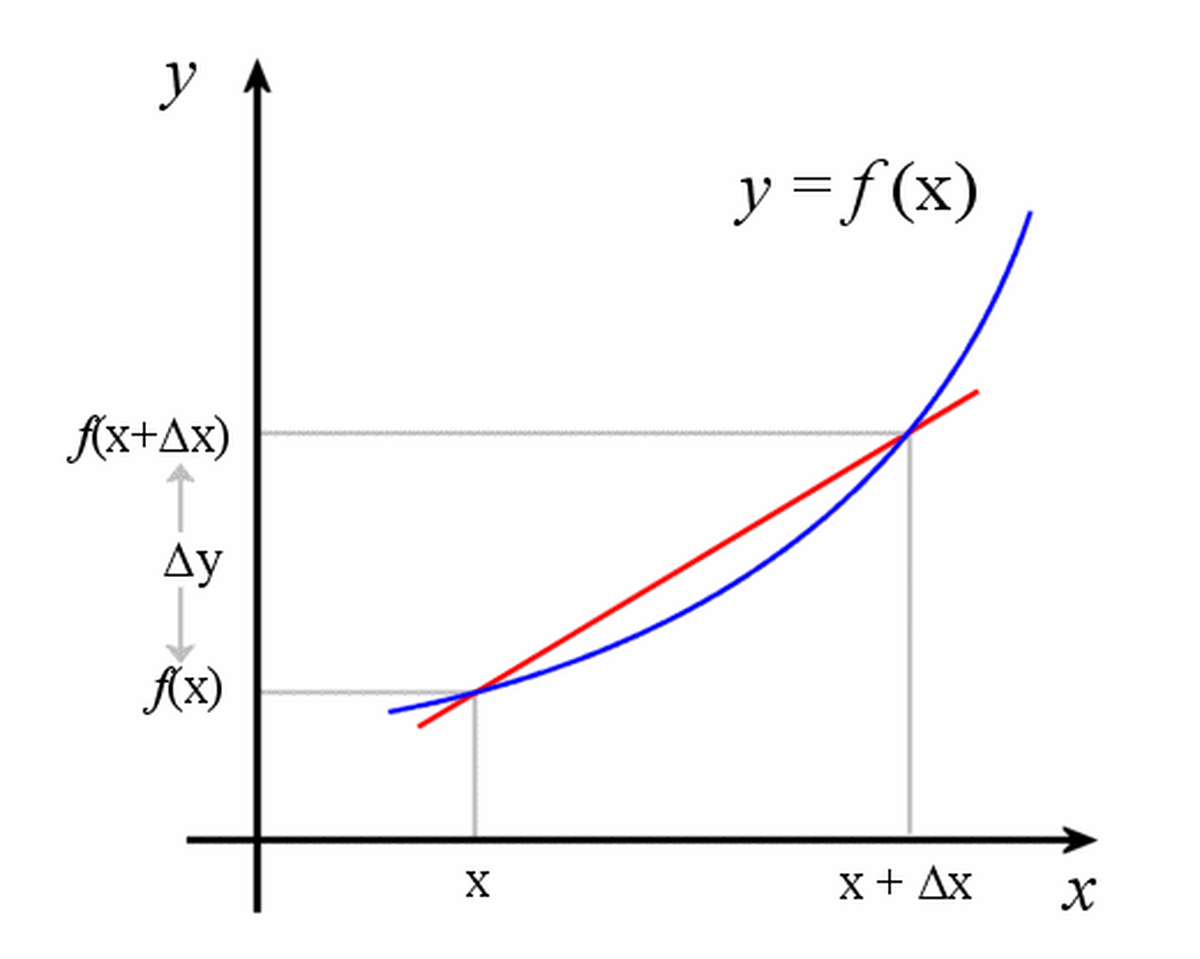As Δx and Δy tend to zero, the slope of the secant approaches the slope of the tangent.© Eugene Brennan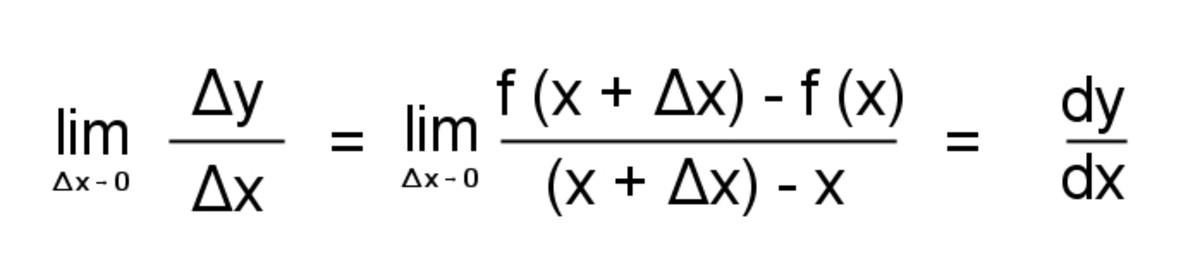Slope over an interval Δx. The limit is the derivative of the function.© Eugene Brennan

## Differentiating Functions From First Principles

To find the derivative of a function, we differentiate it wrt to the independent variable. There are several identities and rules to make this easier, but first let's try to work out an example from first principles.

Example: Evaluate the derivative of x2

So f (x) = x2

f (x + Δx) = (x + Δx)2

d/dx( f (x)) =

lim ( f (x + Δx) - f (x) ) / ((x + Δx) - x)
Δx → 0

Substitute for f (x + Δx) and f (x) giving

lim ( (x + Δx)2 - x2 ) / Δx)
Δx → 0

Expand out (x + Δx)2 giving

lim ( (x2 + 2xΔx + Δx2- x2 ) / Δx)
Δx → 0

The two x2 cancel out which gives:

lim ( (2xΔx + Δx2 ) / Δx)
Δx → 0

Dividing by Δx gives:

lim (2x + Δx )
Δx → 0

= 2x

## Using Rules to Work out Derivatives

Rather than working out the derivatives of functions from first principles, we normally use a set of rules to make things easier.

In the table below, f and g are two functions.

f ' is the derivative of f

g' is the derivative of g

## Rules of Differentiation

Rule TypeFunctionDerivative

Constant factor rule

af

af '

Power rule for polynomials

xᵇ

bxᵇ ⁻ ¹

Sum rule

f + g

f ' + g '

Difference rule

f - g

f ' - g '

Product rule

fg

fg ' + gf '

Reciprocal of a function

1/f

- f ' / f ^2

Quotient rule

f / g

(f 'g - g ' f )/ g ^2

Chain rule (function of a function rule)

f(g) where g is a function of x

f ' (g) g ' (x)

## Derivatives of Common Functions

Function TypeFunctionDerivative

Constant

c

0

Line

mx

m

Line with y axis intercept

mx + c

m

x squared

x ^ 2

2x

x cubed

x ^ 3

3x^2

Square root

x ^ (1/2)

(1/2) x ^ (-1/2)

Reciprocal

1/x

-1/x^2

Exponential function

e^x

e^x

Natural log

ln (x)

1/x

Trigonometric function

sin (x)

cos (x)

Trigonometric function

cos (x)

- sin (x)

Trigonometric function

tan (x)

1 + (tan (x))^2 = (cosec (x))^2

## Examples of Working Out Derivatives

Example 1:

What is the derivative of 20?

Derivative of a constant is 0, so d/dx(20) = 0

Example 2:

What is the derivative of 3x?

Using the constant factor rule (multiplication by a constant rule)

d/dx(3x) = 3( d/dx(x) )

But using the power rule the derivative of x1 = 1x0 = 1

So d/dx(3x) = 3( d/dx(x) ) = 3(1) = 3

Example 3:

What is the derivative of 6x3

Using the multiplication by a constant rule, d/dx(6x3) = 6 ( d/dx(x3) )

From the power rule, d/dx(x3) = 3x2

So d/dx(6x3) = 6 ( d/dx(x3) ) = 6 (3x2) = 18x2

Example 4:

Evaluate the derivative of 5sin (x) + 6x5

We use the sum rule to find the derivatives of 5sin (x) and 6x5 and then add the result together.

So d/dx (5sin (x)) = 5d/dx(sin (x)) = 5cos (x)

d/dx(6x5) = 6d/dx(x5) = 6(5x4) = 30x4

Adding the results together

d/dx (5sin (x) + 6x5) = 5cos (x) + 30x4

Example 5:

What is the derivative of x3sin (x) ?

Use the product rule, so:

d/dx(x3sin (x)) = x3d/dx(sin(x)) + sin(x)d/dx(x3)

d/dx (sin(x)) = cos(x)

d/dx(x3) = 3x2 (from the power rule)

so d/dx(x3sin (x)) = x3d/dx(sin(x)) + sin(x)d/dx(x3) = x3cos(x) + 3x2sin(x)

Example 6:

Evaluate the derivative of tan (x)

tan (x) = sin (x) / cos (x)

We can use the quotient rule to work this out:

d/dx (f(x)/g(x)) = (f '(x)g(x) - g '(x)f (x))/ g(x)2

so d/dx(sin (x) / cos (x)) = (d/dx(sin (x))cos (x) - d/dx(cos (x))sin (x)) / cos2 (x)

d/dx(sin (x)) = cos (x)

d/dx(cos (x)) = - sin x

Substituting

(d/dx(sin (x))cos (x) - d/dx(cos (x))sin (x)) / cos2(x)

= (cos (x)cos (x) - (-sin (x))sin (x)) / cos2(x)

= (cos2(x) + sin2(x)) / cos2(x)

= 1 + tan2(x) = sec2(x)

Example 7:

What is the derivative of ln(5x3) ?

We use the chain rule to work this out.

For two functions f(g) and g(x)

df/dx = (df/dg)(dg/dx)

Let g(x) = 5x3

and f(g) = ln(g)

df/dg = 1/g

dg/dx = 5(3x2) = 15x2

df/dx = (df/dg)(dg/dx)

= (1/g)(15x2)

Substituting for g:

= 1/(5x3)((15x2) = 3/x

We could also have evaluated the derivative by first using the rules of logarithms to simplify the expression.

So ln(5x3) = ln(5) + ln(x3) = ln(5) + 3ln(x) ............(product rule and power rule)

d/dx (ln(5) + 3ln(x)) = d/dx (ln(5)) + d/dx(3ln(x)) = 0 + 3d/dx(ln(x))

= 0 + 3(1/x) = 3/x

## Positive and Negative Values of the Derivative

The animation below shows the function sin(Ө) and it's derivative cos(Ө). At Ө = 0, the value of the derivative is cos(Ө) = cos(0) = 1. As Ө increases, the value of cos(Ө) decreases, i.e the slope of the tangent to sin(Ө) becomes smaller. Eventually at Ө = π/2, the slope is zero. This is an important point because as we'll see later, we can use this fact to find the maxima and minima of functions.
As Ө exceeds π/2, the value of the derivative becomes negative.

How come?

Remember the definition of the derivative?

Δx is positive, but the change Δy is negative since f (x +Δx) - f (x) is negative because f (x +Δx) < f (x)......(the function is decreasing in value)

In the limit as Δx and Δy tend to zero, the derivative is also less than zero.

## Sin(Ө) and Its Derivative Cos(Ө)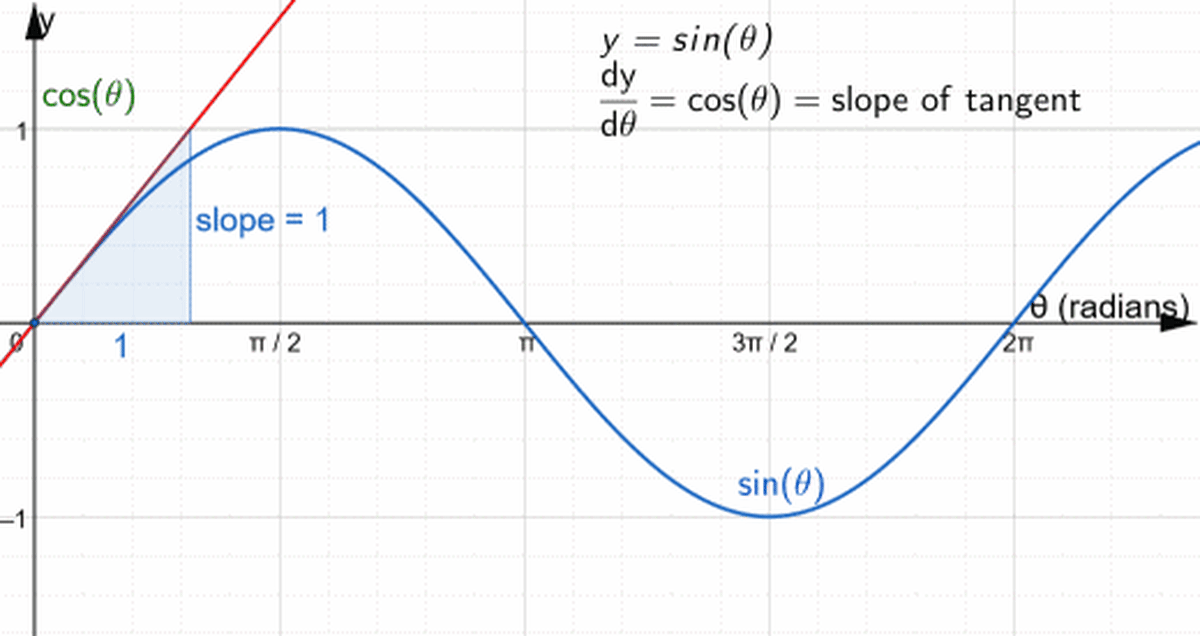The derivative of sin(Ө) is cos(Ө). Notice how the value of the derivative at Ө = 0 is positive and decreases to 0 at the peak of the waveform when Ө = π/2. Then it becomes negative and reaches zero before becoming positive again.© Eugene Brennan - Created in GeoGebra

## 2nd and Higher Order Derivatives

What happens if we take the derivative of a derivative?

Consider the function y = f (x)

The derivative of y is f '(x) or dy/dx

The derivative of dy/dx is known as the second derivative or second order derivative and is denoted by d2y/dx2 or f '' (x) ......(f with a double dash). We can have third and higher order derivatives so for instance the third order derivative of y is d3y/dx3

Examples:

(1) If y = sin (x), what is d2y/dx2 ?

dy/dx = cos (x)

d2y/dx2 = d/dx (cos (x)) = -sin (x)

(2) What is the second derivative of ln (x)?

y = ln (x)

So dy/dx = 1/x = x(-1)

d2y/dx2 = d/dx (x(-1)) = -1( x(-2) ) = -1/x2

Since dy/dx is the rate of change of a function, roughly speaking we can think of d2y/dx2 as being the rate at which dy/dx itself is changing.

Returning to the car example:

s(t) is a function describing how distance travelled changes with time.
ds/dt is the rate of change of position, called speed or velocity.
d2s/dt2 is the rate of change of velocity, which is called acceleration.

If v is the velocity of the vehicle and a is its acceleration:

v = ds/dt

a = dv/dt = d/dt(ds/dt) = d2s/dt2

So the second derivative of distance which is acceleration is equal to the first derivative of velocity.

We can go up to the third derivative of s, so:

d3s/dt3 = da/dt is the rate of change of acceleration, known as "jerk".

## Stationary and Turning Points of a Function

A stationary point of a function is a point at which the derivative is zero. On a graph of the function, the tangent to the point is horizontal and parallel to the x-axis.

A turning point of a function is a point at which the derivative changes sign. A turning point can be either a local maxima or minima. If a function can be differentiated, a turning point is a stationary point. However the reverse is not true. Not all stationary points are turning points. For instance in the graph of f (x) = x3 below, the derivative f '(x) at x = 0 is zero and so x is a stationary point. However as x approaches 0 from the left, the derivative is positive and decreases to zero, but then increases positively as x becomes positive again. Therefore the derivative doesn't change sign and x is not a turning point.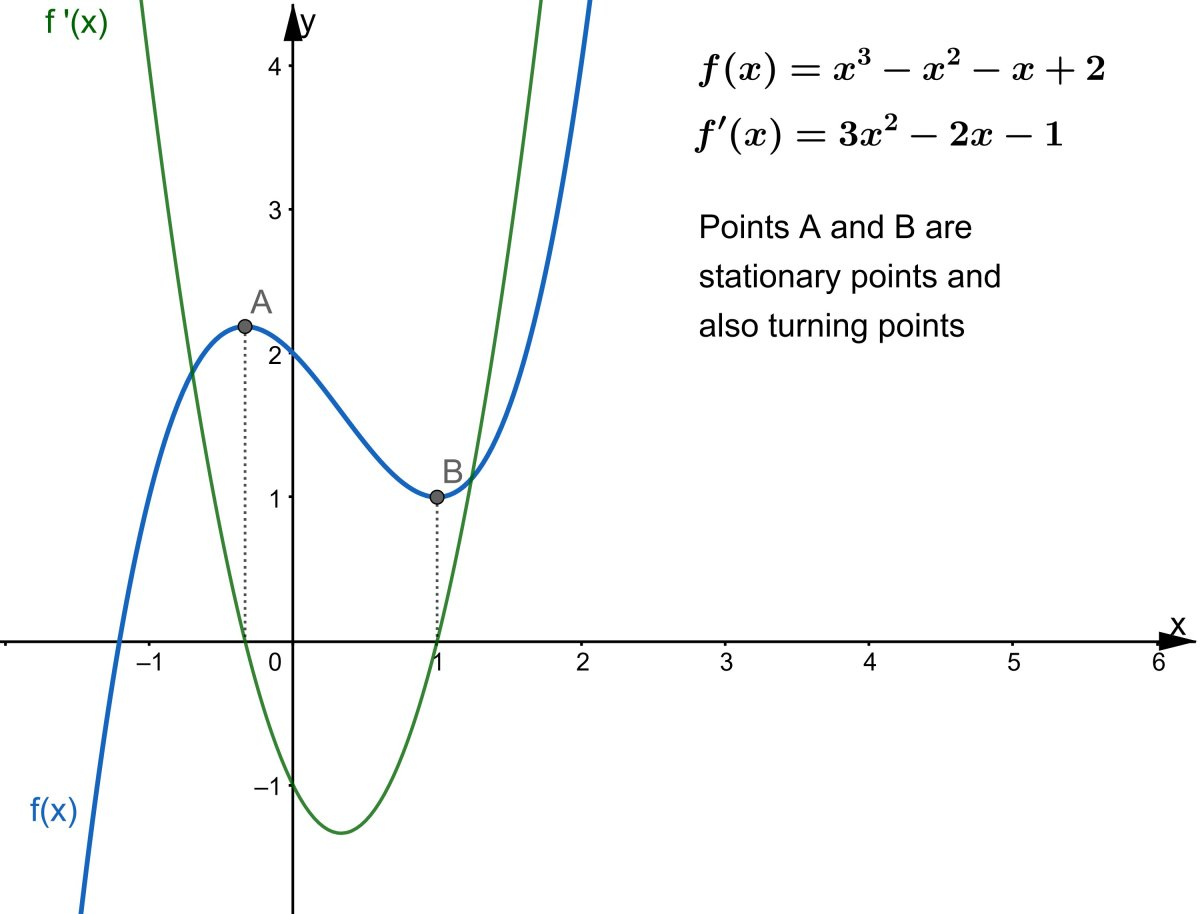Points A and B are stationary points and the derivative f'(x) = 0. They are also turning points because the derivative changes sign.© Eugene Brennan - Created in GeoGebra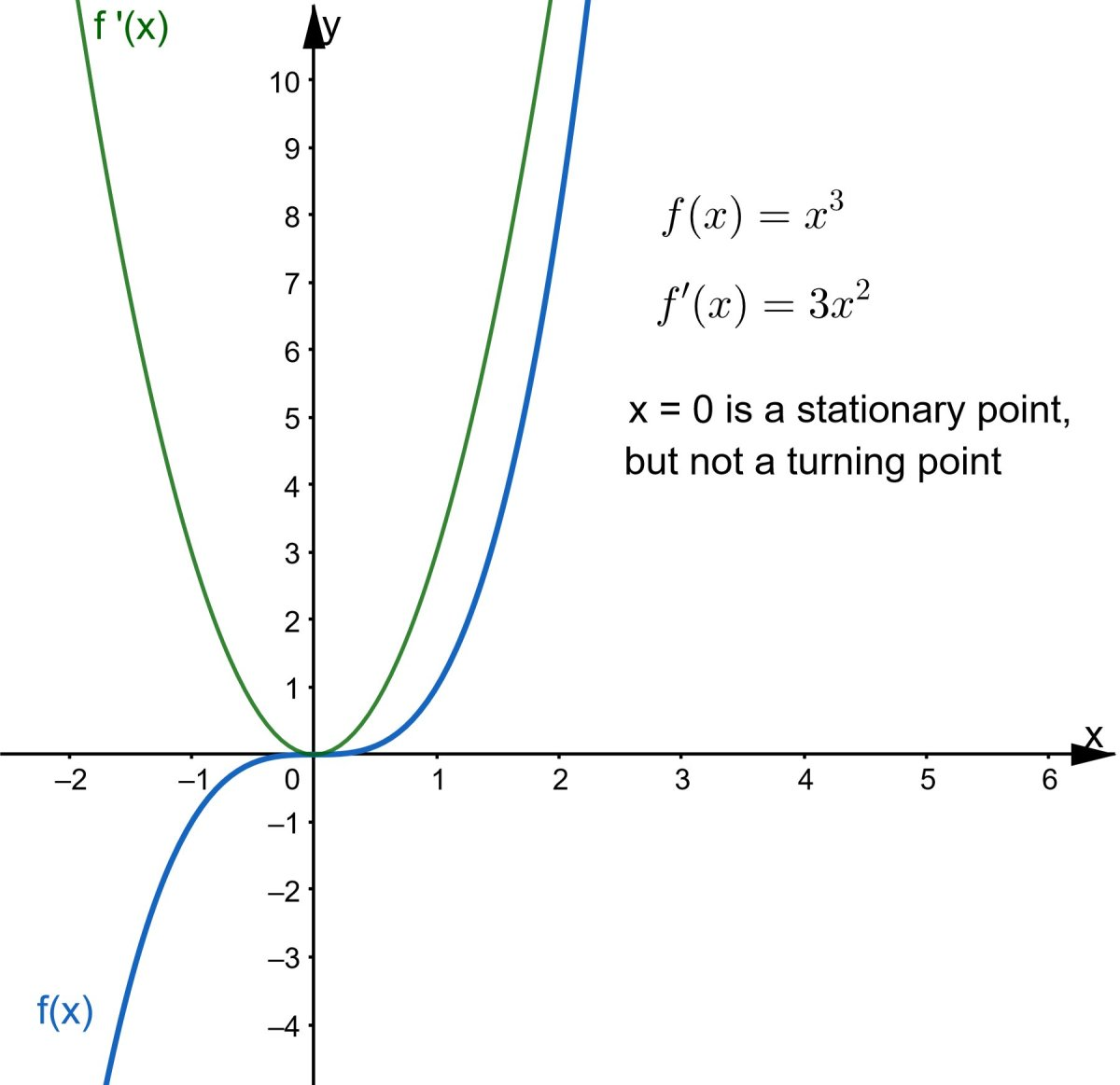Example of a function with a stationary point that is not a turning point. The derivative f'(x) at x = 0 is 0, but doesn't change sign.© Eugene Brennan - Created in GeoGebra

## Inflection Points of a Function

An inflection point of a function is a point on a curve at which the function changes from being concave to convex. At an inflection point, the second order derivative changes sign (i.e it passes through 0. See the graph below for a visualisation).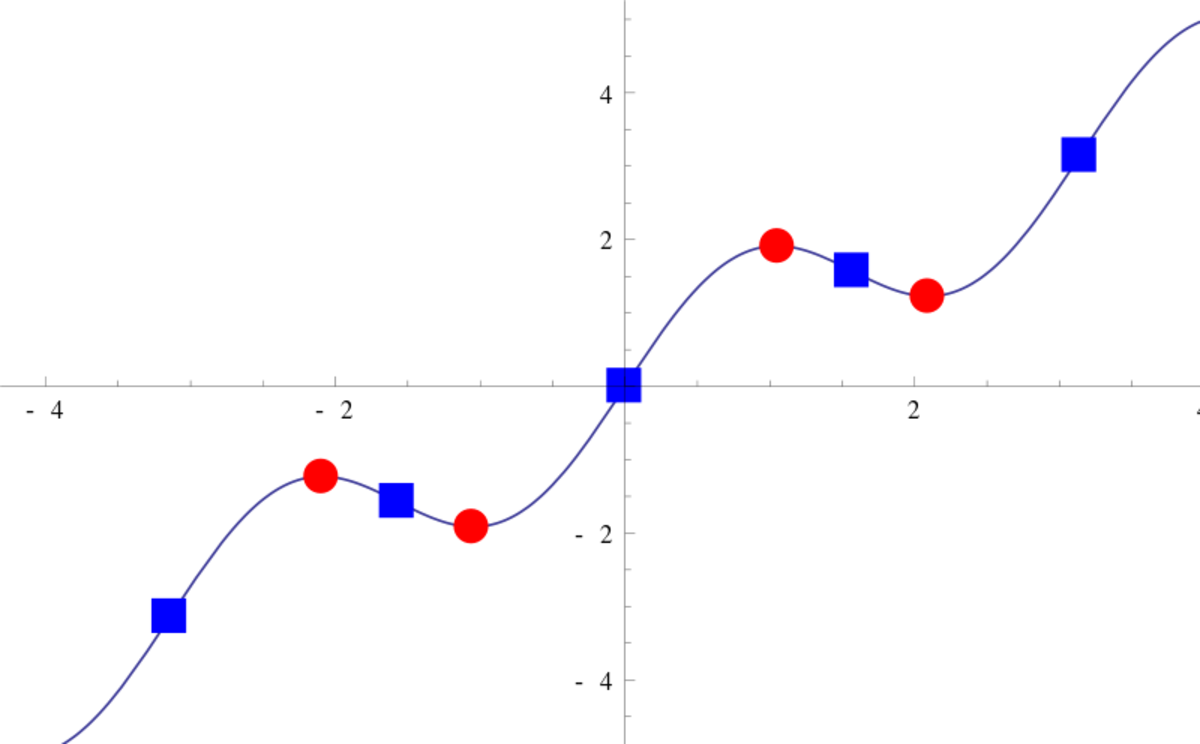The red circles are stationary points. The blue squares are inflection points.Self CC BY SA 3.0 via Wikimedia Commons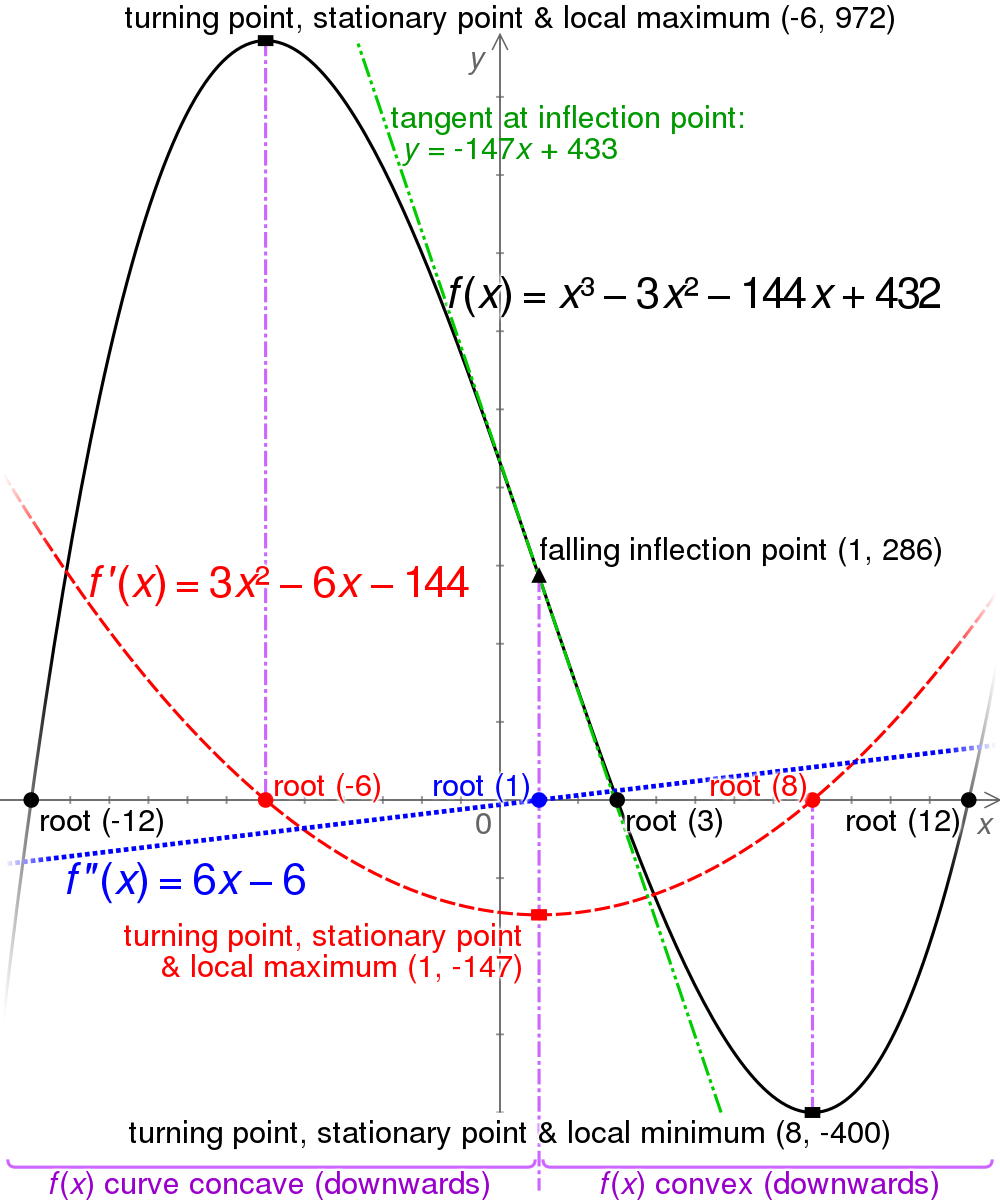Explaining stationary, turning points and inflection points and how they relate to the first and second order derivativesCmglee, CC BY SA 3.0 unported via Wikimedia Commons

## Using the Derivative to Find the Maxima, Minima and Turning Points of Functions

We can use the derivative to find the local maxima and minima of a function (the points at which the function has maximum and minimum values.) These points are called turning points because the derivative changes sign from positive to negative or vice versa. For a function f (x), we do this by:

• differentiating f (x) wrt x
• equating f ' (x) to 0
• and finding the roots of the equation, i.e. the values of x that make f '(x) = 0

Example 1:

Find the maxima or minima of the quadratic function f (x) = 3x2 + 2x +7 (the graph of a quadratic function is called a parabola).

f (x) = 3x2 + 2x +7

and f '(x) = 3(2x1) + 2(1x0) + 0 = 6x + 2

Set f '(x) = 0

6x + 2 = 0

Solve 6x + 2 = 0

Rearranging:

6x = -2

giving x = - 1/3

and f(x) = 3x2 + 2x +7 = 3(-1/3)2 + 2(-1/3) + 7 = 6 2/3

A quadratic function has a maximum when the coefficient of x² < 0 and a minimum when the coefficient > 0. In this case since the coefficient of x² was 3, the graph "opens up" and we have worked out the minimum and it occurs at the point (- 1/3, 6 2/3).

Example 2:

In the diagram below, a looped piece of string of length p is stretched into the shape of a rectangle. The sides of the rectangle are of length a and b. Depending on how the string is arranged, a and b can be varied and different areas of rectangle can be enclosed by the string. What is the maximum area that can be enclosed and what will be the relationship between a and b in this scenario?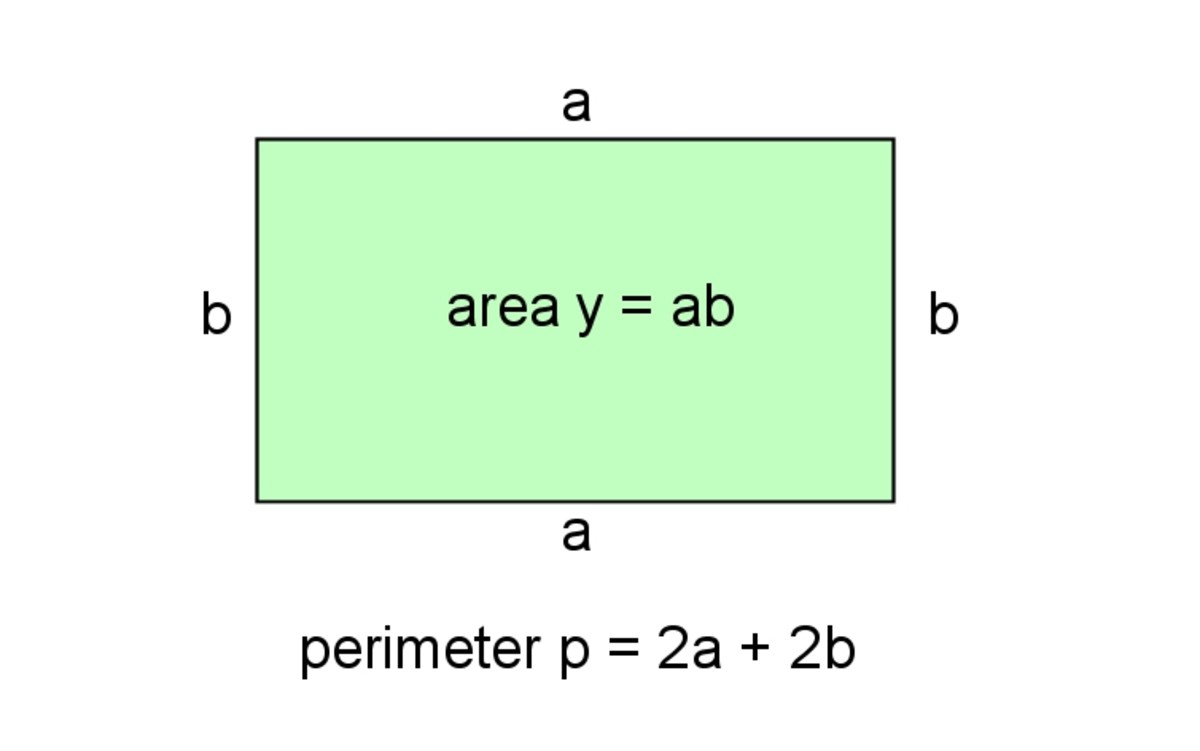Finding the maximum area of a rectangle that can be enclosed by a perimeter of fixed length© Eugene Brennan

p is the length of the string

The perimeter p = 2a + 2b (the sum of the 4 side lengths)

Call the area y

and y = ab

We need to find an equation for y in terms of one of the sides a or b, so we need to eliminate either of these variables.

Let's try to find b in terms of a:

So p = 2a + 2b

Rearranging:

2b = p - 2a

and:

b = (p - 2a)/2

y = ab

Substituting for b gives:

y = ab = a(p - 2a)/2 = ap/2 - a2 = (p/2)a - a2

Work out the derivative dy/da and set it to 0 (p is a constant):

dy/da = d/da((p/2)a - a2) = p/2 - 2a

Set to 0:

p/2 - 2a = 0

Rearranging:

2a = p/2

so a = p/4

We can use the perimeter equation to work out b, but it's obvious that if a = p/4 the opposite side is p/4, so the two sides together make up half the length of the string which means both of the other sides together are half the length. In other words maximum area occurs when all sides are equal. I.e when the enclosed area is a square.

So area y = (p/4)(p/4) = p2/16

Example 3 (Max Power Transfer Theorem or Jacobi's Law):

The image below shows the simplified electrical schematic of a power supply. All power supplies have an internal resistance (RINT) which limits how much current they can supply to a load (RL). Calculate in terms of RINT the value of RL at which maximum power transfer occurs.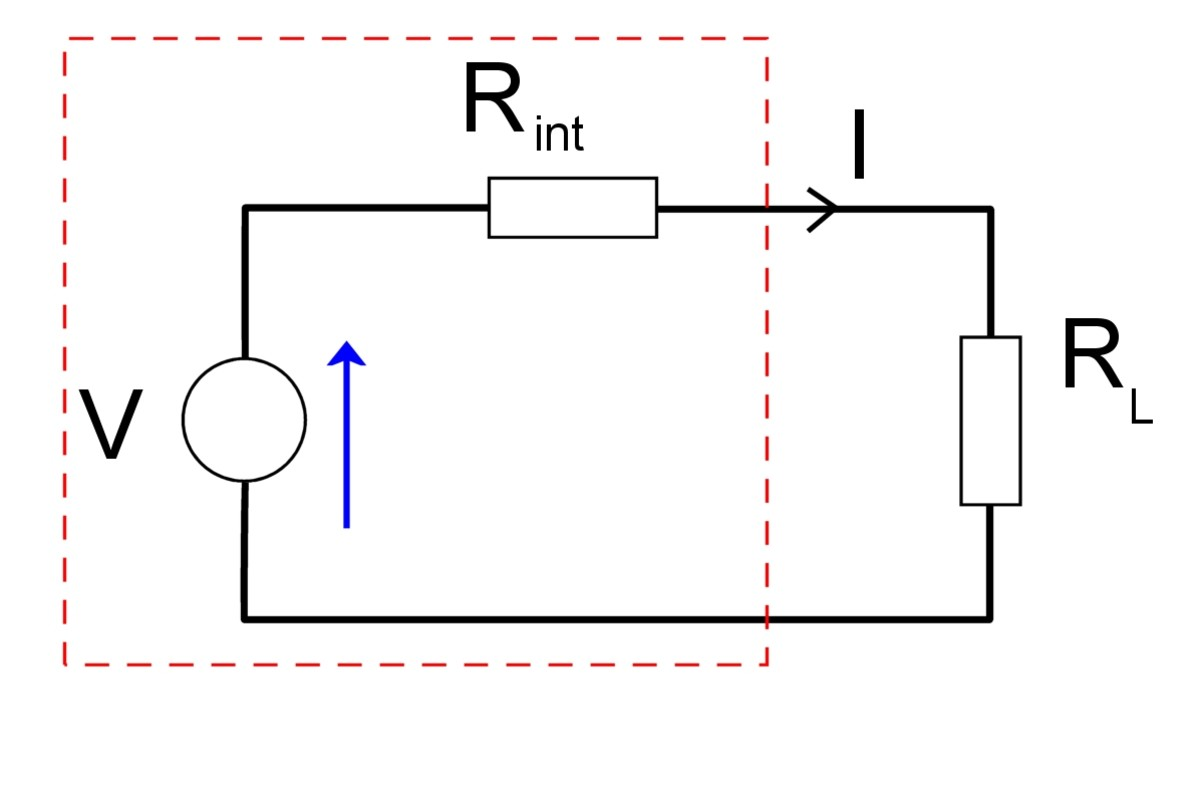The schematic of a power supply connected to a load, showing the supply's equivalent internal resistance Rint© Eugene Brennan

The current I through the circuit is given by Ohm's Law:

So I = V/(RINT+ RL)

Power = Current squared x resistance

So power dissipated in the load RL is given by the expression:

P = I2RL

Substituting for I:

= (V/(RINT+ RL))2RL

= V2RL/(RINT+ RL)2

Expanding the denominator:

= V2RL/(R2INT + 2RINTRL + R2L)

and dividing above and below by RL gives:

P = V2 / (R2INT / RL + 2RINT + RL)

Rather than finding when this is a maximum, it's easier to find when the denominator is a minimum and this gives us the point at which maximum power transfer occurs, i.e. P is a maximum.

So the denominator is R2INT / RL + 2RINT + RL

Differentiate it wrt RL giving:

d/dRL (R2INT / RL + 2RINT + RL) = -R2INT / R2L + 0 + 1

Set it to 0:

-R2INT / R2L + 0 + 1 = 0

Rearranging:

R2INT / R2L = 1

and solving gives RL = RINT.

So max power transfer occurs when RL = RINT.

This is called the max power transfer theorem.

Engineering Mathematics by K.A. Stroud, available on Amazon, is an excellent math textbook for both engineering students and anyone with a general interest in mathematics. The material has been written for part 1 of BSc. Engineering Degrees and Higher National Diploma courses.

A wide range of topics are covered, including matrices, vectors, complex numbers, calculus, calculus applications, differential equations and series. The text is written in the style of a personal tutor, guiding the reader through the content, posing questions and encouraging them to provide the answer. Personally, I've found it really easy to follow.

It also covers a more in-depth treatment of probability theory than what has been covered in this article, plus a section on statistics.

This book basically makes learning mathematics fun!

Note: Second-hand 1987 editions of this textbook are available on Amazon for about \$20.

## A Beginner's Guide to Integration

See my other tutorial, which covers integral calculus and applications of integration:

How to Understand Calculus: A Beginner's Guide to Integration

Stroud, K.A., (1970) Engineering Mathematics (3rd ed., 1987) Macmillan Education Ltd., London, England.

This content is accurate and true to the best of the author’s knowledge and is not meant to substitute for formal and individualized advice from a qualified professional.

© 2019 Eugene Brennan

Eugene Brennan (author) from Ireland on January 26, 2020:

Hi Andrey,

Yes you're right, if the coefficient of x² is positive, the graph "opens" up and it has a minima. If the coefficient is negative, the graph "opens down" and it has a maximum.

Thanks for spotting the mistake.

I've explained this in another tutorial:

https://owlcation.com/stem/How-to-Understand-the-E...

Andrey Pustoshkin on January 26, 2020:

A quadratic function has a maximum when the coefficient of x - 0 and a minimum when the coefficient + 0. In this case since the coefficient of x was 2

maybe maximum when coeff of x^2 - 0, ... coef of x^2 was 3? or i misunderstanding?

Alice Mavhunex on December 07, 2019:

great

bablu bhattacharjee on September 24, 2019:

Excellent.